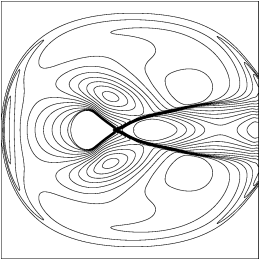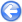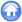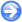### 13.1PASS: Circular dam break on a sphere

Author
Stéphane Popinet
Command
gerris2D -m lonlat.gfs
Version
090924
Required files
isolines.gfv
Running time
3 minutes 2 seconds

An initial circular cylinder collapses and creates shock and rarefaction waves. The initial condition are radially-symmetric and should remain so. The problem is discretised using longitude-latitude spherical coordinates. Deviations from radial symmetry are a measure of the accuracy of treatment of geometric source terms.

This test case was proposed by , Figures 5 and 6.

 Figure 156: Solution to the shallow-water equations computed on a longitude-latitude grid in the domain [−75∘,75∘]×[−75∘,75∘] with 256× 256 points. The solution is shown at times (a) t=0.3, (b) t=0.6, and (c) t=0.9. The contours do not appear circular because the solution has been projected down to a plane.
 (a)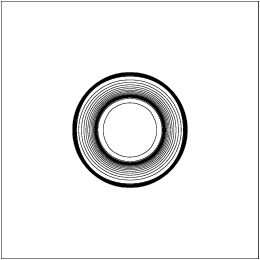(b)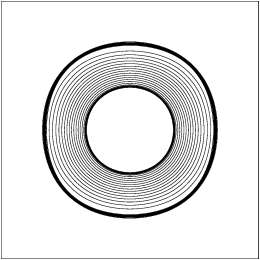(c)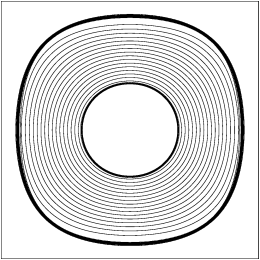Figure 157: Scatter plot of the (radial) solution shown in Figure 156. The green line is the average solution. The solution is shown at times (a) t=0.3, (b) t=0.6, and (c) t=0.9.
 (a)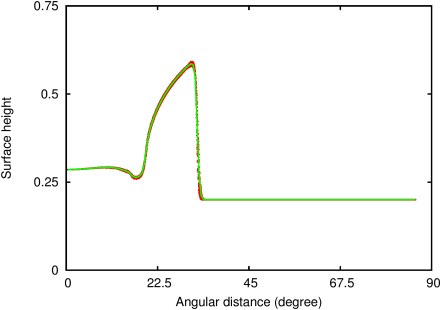(b)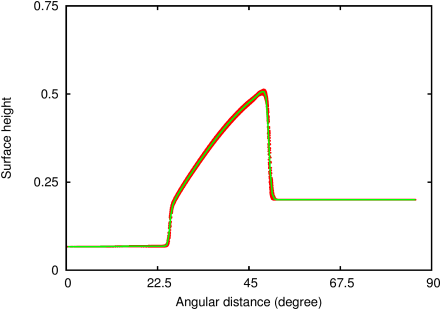(c)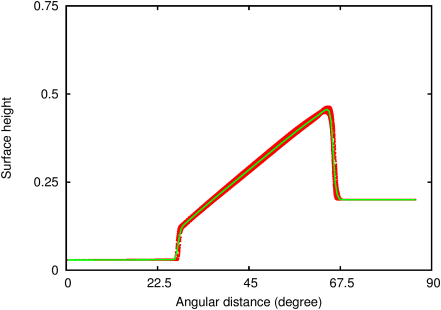#### 13.1.1PASS: Circular dam break on a “cubed sphere”

Author
Stéphane Popinet
Command
gerris2D -m cubed.gfs
Version
120812
Required files
isolines.gfv
Running time
8 minutes 24 seconds

Same test case but using a “cubed sphere” metric and adaptive mesh refinement. There is noticeable distortion close to the cubed sphere “poles” (Figures 158.(c) and 159.(c)).

 Figure 158: Solution to the shallow-water equations computed on a “cubed sphere” with adaptive mesh refinement. The solution is shown at times (a) t=0.3, (b) t=0.6, (c) t=0.9, (d) t=1.2, and (e) t=1.5. The contours do not appear circular because the solution has been projected down to a plane.
 (a)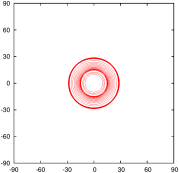(b)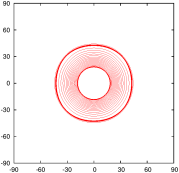(c)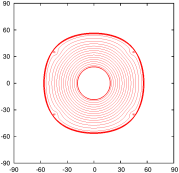(d)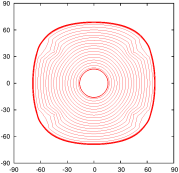(e)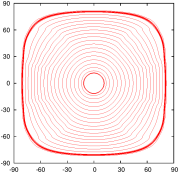Figure 159: Scatter plot of the (radial) solution shown in Figure 158. The green line is the average solution. The solution is shown at times (a) t=0.3, (b) t=0.6, (c) t=0.9, (d) t=1.2, and (e) t=1.5.
 (a)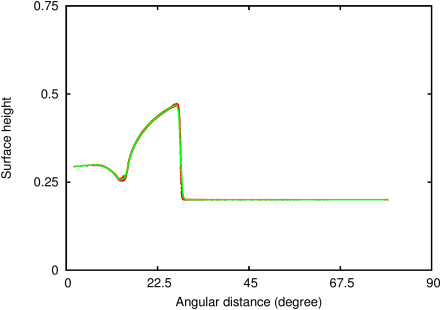(b)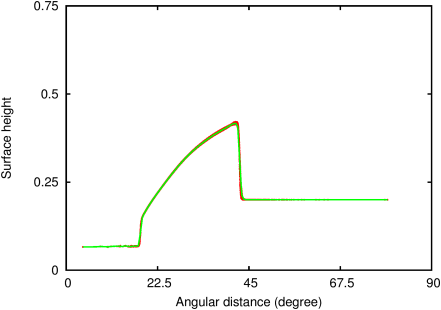(c)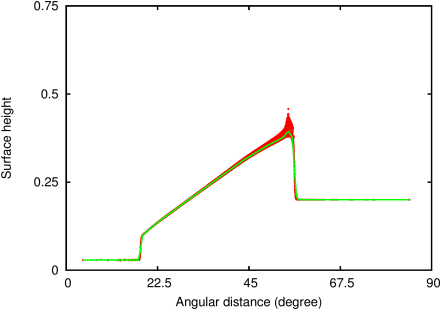(d)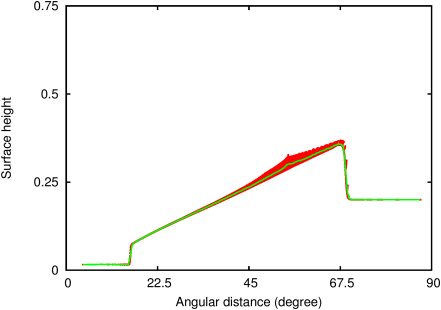(e)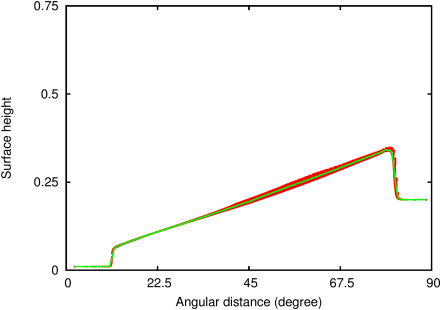#### 13.1.2PASS: Circular dam break on a rotating sphere

Author
Stéphane Popinet
Command
gerris2D -m coriolis.gfs
Version
090924
Required files
 (a)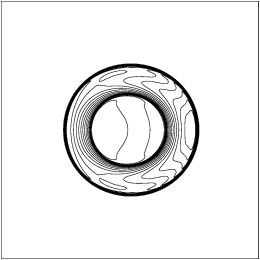(b)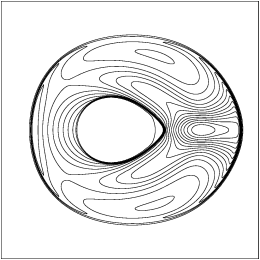(c)